# RS Aggarwal Solutions for Class 7 Maths Chapter 12 Simple Interest

RS Aggarwal Solutions for Class 7 Maths Chapter 12 – Simple Interest are available here. Students can check for the solutions whenever they are facing difficulty, while solving the questions from RS Aggarwal Class 7 Solutions. These solutions for chapter 12 are available in PDF format so that students can download it and learn offline as well. This book is one of the top materials when it comes to providing a question bank to practice from.

We at BYJU’S have prepared the RS Aggarwal Solutions for Class 7 Maths Chapter 12 wherein, problems are formulated by our expert tutors to assist you with your exam preparation to attain good marks in Maths. Download pdf of Class 7 Chapter 12 in their respective links.

## Download PDF of RS Aggarwal Solutions for Class 7 Maths Chapter 12 – Simple Interest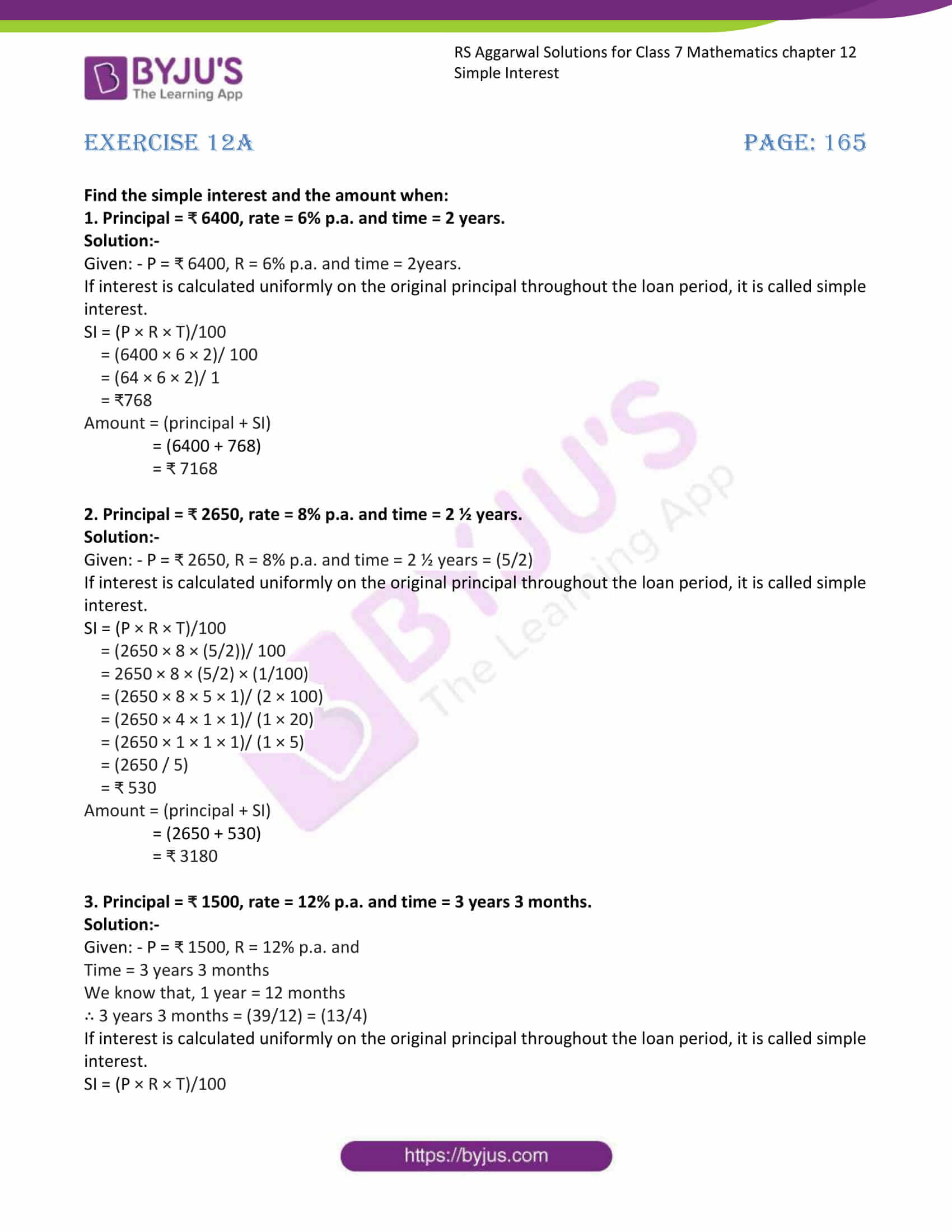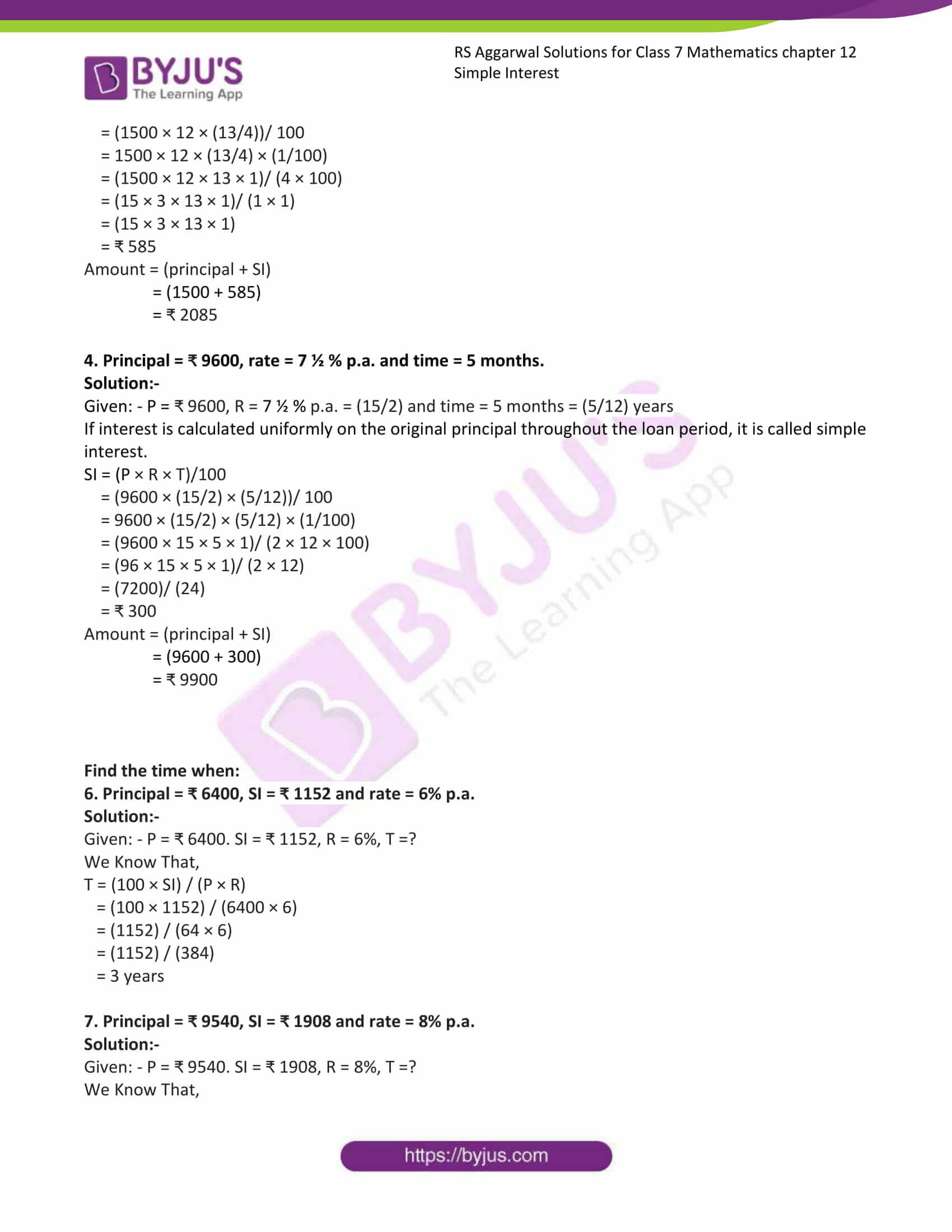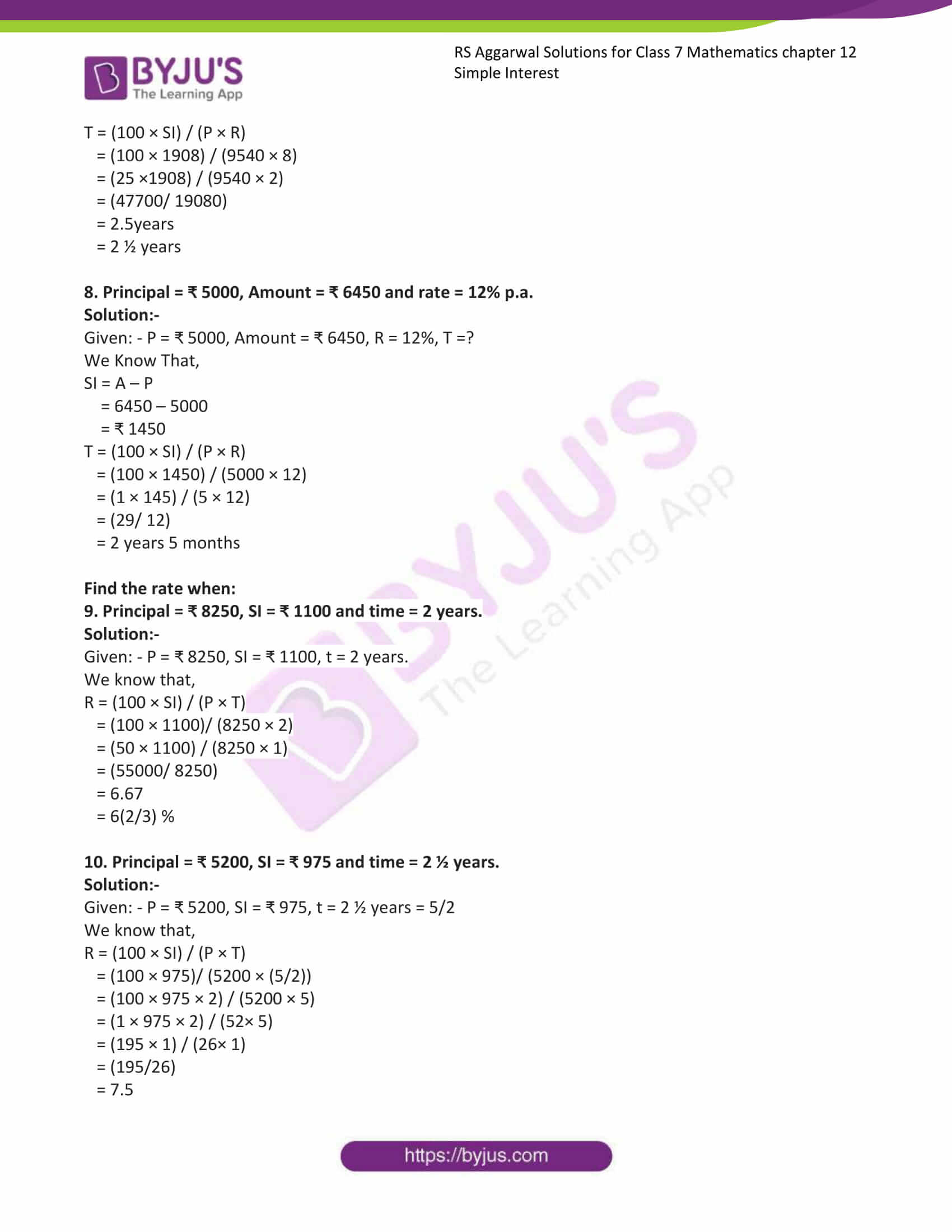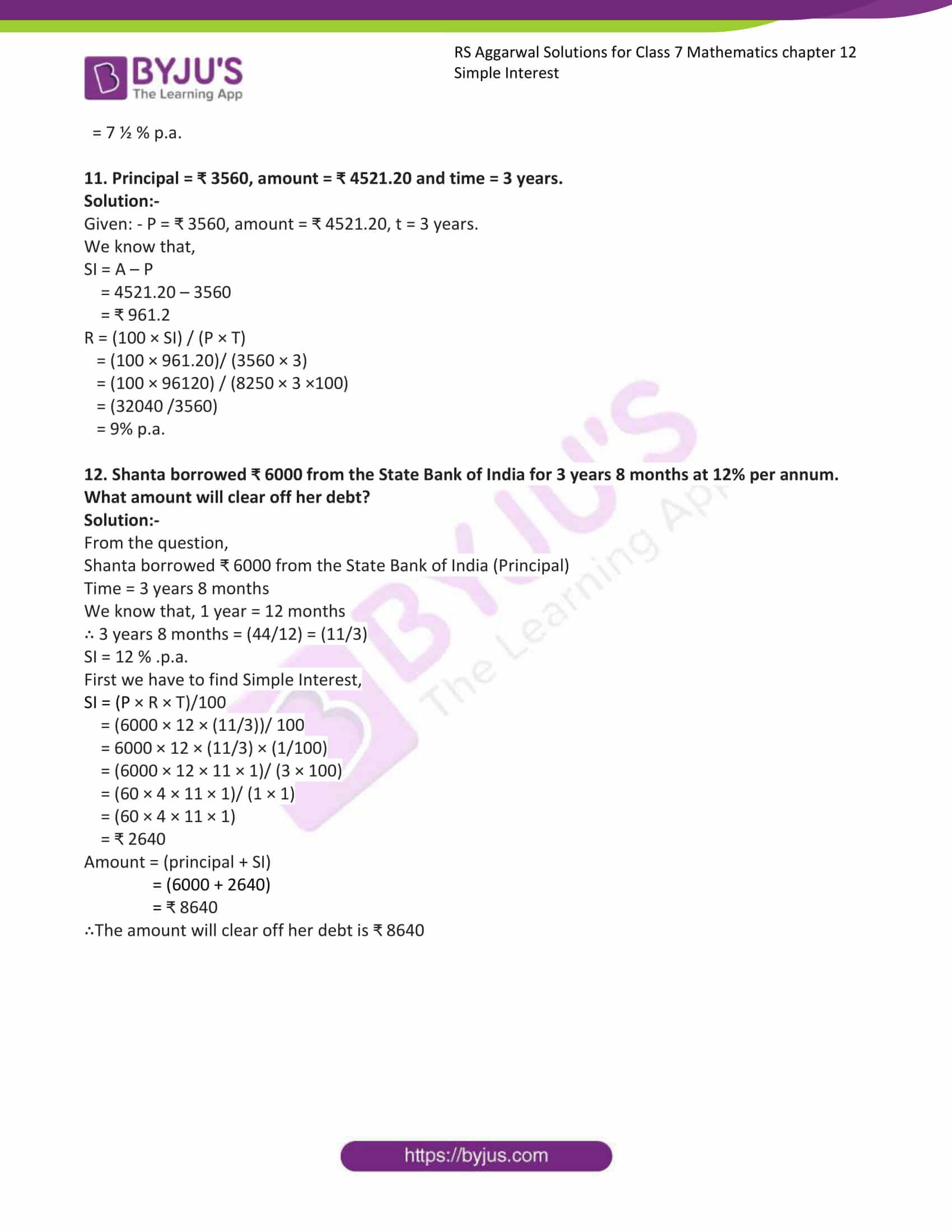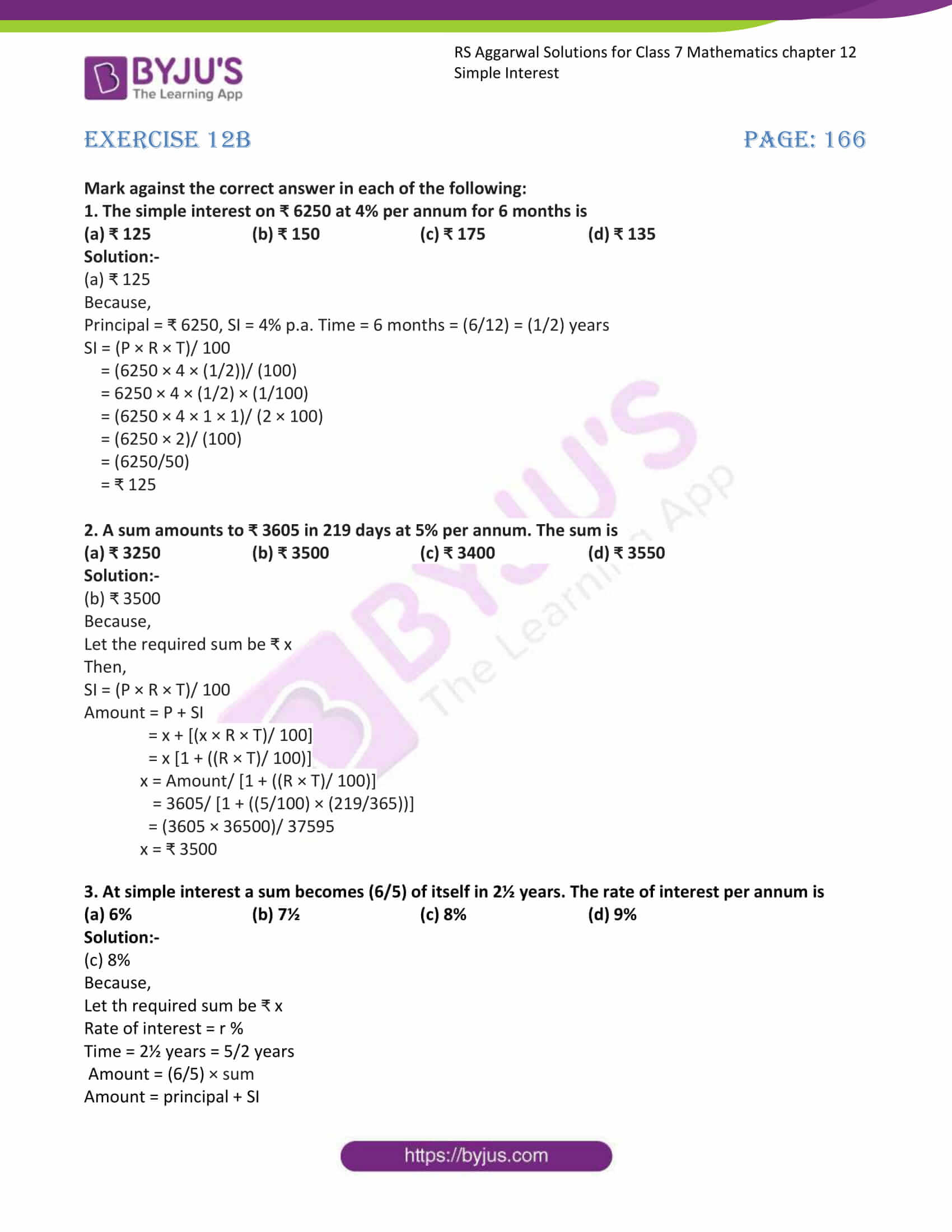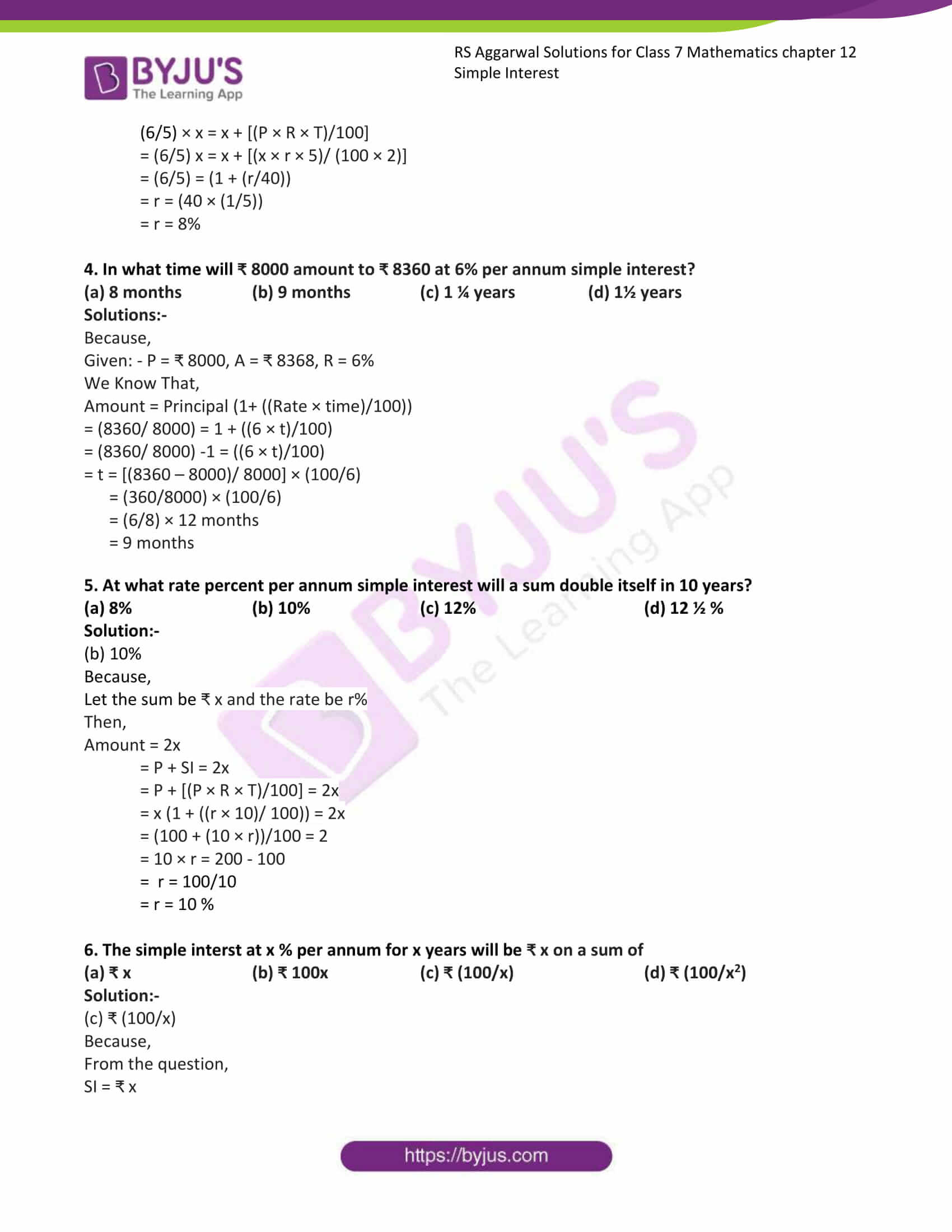### Also, access RS Aggarwal Solutions for Class 7 Chapter 12 Exercises

Exercise 12A

Exercise 12B

Exercise 12a

Find the simple interest and the amount when:

1. Principal = ₹ 6400, rate = 6% p.a. and time = 2 years.

Solution:-

Given: – P = ₹ 6400, R = 6% p.a. and time = 2years.

If interest is calculated uniformly on the original principal throughout the loan period, it is called simple interest.

SI = (P × R × T)/100

= (6400 × 6 × 2)/ 100

= (64 × 6 × 2)/ 1

= ₹768

Amount = (principal + SI)

= (6400 + 768)

= ₹ 7168

2. Principal = ₹ 2650, rate = 8% p.a. and time = 2 ½ years.

Solution:-

Given: – P = ₹ 2650, R = 8% p.a. and time = 2 ½ years = (5/2)

If interest is calculated uniformly on the original principal throughout the loan period, it is called simple interest.

SI = (P × R × T)/100

= (2650 × 8 × (5/2))/ 100

= 2650 × 8 × (5/2) × (1/100)

= (2650 × 8 × 5 × 1)/ (2 × 100)

= (2650 × 4 × 1 × 1)/ (1 × 20)

= (2650 × 1 × 1 × 1)/ (1 × 5)

= (2650 / 5)

= ₹ 530

Amount = (principal + SI)

= (2650 + 530)

= ₹ 3180

3. Principal = ₹ 1500, rate = 12% p.a. and time = 3 years 3 months.

Solution:-

Given: – P = ₹ 1500, R = 12% p.a. and

Time = 3 years 3 months

We know that, 1 year = 12 months

∴ 3 years 3 months = (39/12) = (13/4)

If interest is calculated uniformly on the original principal throughout the loan period, it is called simple interest.

SI = (P × R × T)/100

= (1500 × 12 × (13/4))/ 100

= 1500 × 12 × (13/4) × (1/100)

= (1500 × 12 × 13 × 1)/ (4 × 100)

= (15 × 3 × 13 × 1)/ (1 × 1)

= (15 × 3 × 13 × 1)

= ₹ 585

Amount = (principal + SI)

= (1500 + 585)

= ₹ 2085

4. Principal = ₹ 9600, rate = 7 ½ % p.a. and time = 5 months.

Solution:-

Given: – P = ₹ 9600, R = 7 ½ % p.a. = (15/2) and time = 5 months = (5/12) years

If interest is calculated uniformly on the original principal throughout the loan period, it is called simple interest.

SI = (P × R × T)/100

= (9600 × (15/2) × (5/12))/ 100

= 9600 × (15/2) × (5/12) × (1/100)

= (9600 × 15 × 5 × 1)/ (2 × 12 × 100)

= (96 × 15 × 5 × 1)/ (2 × 12)

= (7200)/ (24)

= ₹ 300

Amount = (principal + SI)

= (9600 + 300)

= ₹ 9900

Find the time when:

6. Principal = ₹ 6400, SI = ₹ 1152 and rate = 6% p.a.

Solution:-

Given: – P = ₹ 6400. SI = ₹ 1152, R = 6%, T =?

We Know That,

T = (100 × SI) / (P × R)

= (100 × 1152) / (6400 × 6)

= (1152) / (64 × 6)

= (1152) / (384)

= 3 years

7. Principal = ₹ 9540, SI = ₹ 1908 and rate = 8% p.a.

Solution:-

Given: – P = ₹ 9540. SI = ₹ 1908, R = 8%, T =?

We Know That,

T = (100 × SI) / (P × R)

= (100 × 1908) / (9540 × 8)

= (25 ×1908) / (9540 × 2)

= (47700/ 19080)

= 2.5years

= 2 ½ years

8. Principal = ₹ 5000, Amount = ₹ 6450 and rate = 12% p.a.

Solution:-

Given: – P = ₹ 5000, Amount = ₹ 6450, R = 12%, T =?

We Know That,

SI = A – P

= 6450 – 5000

= ₹ 1450

T = (100 × SI) / (P × R)

= (100 × 1450) / (5000 × 12)

= (1 × 145) / (5 × 12)

= (29/ 12)

= 2 years 5 months

Find the rate when:

9. Principal = ₹ 8250, SI = ₹ 1100 and time = 2 years.

Solution:-

Given: – P = ₹ 8250, SI = ₹ 1100, t = 2 years.

We know that,

R = (100 × SI) / (P × T)

= (100 × 1100)/ (8250 × 2)

= (50 × 1100) / (8250 × 1)

= (55000/ 8250)

= 6.67

= 6(2/3) %

10. Principal = ₹ 5200, SI = ₹ 975 and time = 2 ½ years.

Solution:-

Given: – P = ₹ 5200, SI = ₹ 975, t = 2 ½ years = 5/2

We know that,

R = (100 × SI) / (P × T)

= (100 × 975)/ (5200 × (5/2))

= (100 × 975 × 2) / (5200 × 5)

= (1 × 975 × 2) / (52× 5)

= (195 × 1) / (26× 1)

= (195/26)

= 7.5

= 7 ½ % p.a.

11. Principal = ₹ 3560, amount = ₹ 4521.20 and time = 3 years.

Solution:-

Given: – P = ₹ 3560, amount = ₹ 4521.20, t = 3 years.

We know that,

SI = A – P

= 4521.20 – 3560

= ₹ 961.2

R = (100 × SI) / (P × T)

= (100 × 961.20)/ (3560 × 3)

= (100 × 96120) / (8250 × 3 ×100)

= (32040 /3560)

= 9% p.a.

12. Shanta borrowed ₹ 6000 from the State Bank of India for 3 years 8 months at 12% per annum. What amount will clear off her debt?

Solution:-

From the question,

Shanta borrowed ₹ 6000 from the State Bank of India (Principal)

Time = 3 years 8 months

We know that, 1 year = 12 months

∴ 3 years 8 months = (44/12) = (11/3)

SI = 12 % .p.a.

First we have to find Simple Interest,

SI = (P × R × T)/100

= (6000 × 12 × (11/3))/ 100

= 6000 × 12 × (11/3) × (1/100)

= (6000 × 12 × 11 × 1)/ (3 × 100)

= (60 × 4 × 11 × 1)/ (1 × 1)

= (60 × 4 × 11 × 1)

= ₹ 2640

Amount = (principal + SI)

= (6000 + 2640)

= ₹ 8640

∴The amount will clear off her debt is ₹ 8640

Exercise 12B

Mark against the correct answer in each of the following:

1. The simple interest on ₹ 6250 at 4% per annum for 6 months is

(a) ₹ 125 (b) ₹ 150 (c) ₹ 175 (d) ₹ 135

Solution:-

(a) ₹ 125

Because,

Principal = ₹ 6250, SI = 4% p.a. Time = 6 months = (6/12) = (1/2) years

SI = (P × R × T)/ 100

= (6250 × 4 × (1/2))/ (100)

= 6250 × 4 × (1/2) × (1/100)

= (6250 × 4 × 1 × 1)/ (2 × 100)

= (6250 × 2)/ (100)

= (6250/50)

= ₹ 125

2. A sum amounts to ₹ 3605 in 219 days at 5% per annum. The sum is

(a) ₹ 3250 (b) ₹ 3500 (c) ₹ 3400 (d) ₹ 3550

Solution:-

(b) ₹ 3500

Because,

Let the required sum be ₹ x

Then,

SI = (P × R × T)/ 100

Amount = P + SI

= x + [(x × R × T)/ 100]

= x [1 + ((R × T)/ 100)]

x = Amount/ [1 + ((R × T)/ 100)]

= 3605/ [1 + ((5/100) × (219/365))]

= (3605 × 36500)/ 37595

x = ₹ 3500

3. At simple interest a sum becomes (6/5) of itself in 2½ years. The rate of interest per annum is

(a) 6% (b) 7½ (c) 8% (d) 9%

Solution:-

(c) 8%

Because,

Let th required sum be ₹ x

Rate of interest = r %

Time = 2½ years = 5/2 years

Amount = (6/5) × sum

Amount = principal + SI

(6/5) × x = x + [(P × R × T)/100]

= (6/5) x = x + [(x × r × 5)/ (100 × 2)]

= (6/5) = (1 + (r/40))

= r = (40 × (1/5))

= r = 8%

4. In what time will ₹ 8000 amount to ₹ 8360 at 6% per annum simple interest?

(a) 8 months (b) 9 months (c) 1 ¼ years (d) 1½ years

Solutions:-

Because,

Given: – P = ₹ 8000, A = ₹ 8368, R = 6%

We Know That,

Amount = Principal (1+ ((Rate × time)/100))

= (8360/ 8000) = 1 + ((6 × t)/100)

= (8360/ 8000) -1 = ((6 × t)/100)

= t = [(8360 – 8000)/ 8000] × (100/6)

= (360/8000) × (100/6)

= (6/8) × 12 months

= 9 months

5. At what rate percent per annum simple interest will a sum double itself in 10 years?

(a) 8% (b) 10% (c) 12% (d) 12 ½ %

Solution:-

(b) 10%

Because,

Let the sum be ₹ x and the rate be r%

Then,

Amount = 2x

= P + SI = 2x

= P + [(P × R × T)/100] = 2x

= x (1 + ((r × 10)/ 100)) = 2x

= (100 + (10 × r))/100 = 2

= 10 × r = 200 – 100

= r = 100/10

= r = 10 %

6. The simple interst at x % per annum for x years will be ₹ x on a sum of

(a) ₹ x (b) ₹ 100x (c) ₹ (100/x) (d) ₹ (100/x2)

Solution:-

(c) ₹ (100/x)

Because,

From the question,

SI = ₹ x

Rate = x % .p.a.

Time = x years

Then,

SI = (P × R × T)/ 100

X = (P × X× X)/ 100

P = (100X)/ (X × X)

P = ₹ (100/X)

## RS Aggarwal Solutions for Class 7 Maths Chapter 12 – Simple Interest

Chapter 12 – Simple Interest contains 2 exercises and the RS Aggarwal Solutions available on this page provide solutions to the questions present in the exercises. Now, let us have a look at some of the concepts discussed in this chapter.

• Principal
• Interest
• Amount
• Rate

### Chapter Brief of RS Aggarwal Solutions for Class 7 Maths Chapter 12 – Simple Interest

RS Aggarwal Solutions for Class 7 Maths Chapter 12 – Simple Interest. The money borrowed is the principal. The additional money paid by the borrower is the interest. The total money paid by the borrower to the lender is the amount. These are the topics covered in this chapter along with some word problems.